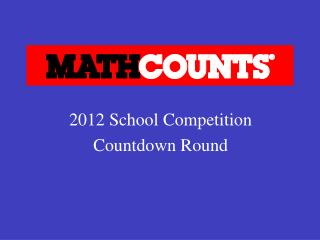DownloadDownload Presentation2012 School Competition Countdown Round

# 2012 School Competition Countdown Round

Download Presentation## 2012 School Competition Countdown Round

- - - - - - - - - - - - - - - - - - - - - - - - - - - E N D - - - - - - - - - - - - - - - - - - - - - - - - - - -
##### Presentation Transcript

1. 2012 School Competition Countdown Round

2. 1. What is the reciprocal of the sum of the reciprocals of 4 and 5? Express your answer as a common fraction.

4. 2. If = 1, then what is the value of x − y?

6. 3. If Mary’s first three test scores are 95, 88 and 100, what score must she make on the next test to have a test average of 90?

8. 4. Luther began reading a 200­page book at 1:15 pm, and he had read 40 pages by 2:00 pm. If he continues to read at the same rate, how many minutes will it take him to read the remainder of the book?

10. 5. The smallest angle of a triangle measures 18˚. What would the degree measure of the smallest angle of the triangle be if the length of each side were doubled?

12. 6. If Lex slices a pie into ten congruent pieces and then eats three pieces, what percent of the pie is left?

14. 7. How many different positive three-digit integers can be formed if the three digits 3, 4 and 5 must be used in each of the integers?

16. 8. Fifteen unit squares are arranged in a 5 by 3 rectangle as shown. What percent of the largest rectangle is shaded gray?

18. 9. What number multiplied by 7 is equal to one more than itself? Express your answer as a common fraction.

20. 10. If a − s − c = −t − b and s = t, what is the value of a + b? Express your answer in terms of c.

22. 11. Jon ran three laps around the school track. He completed the first lap in 5 minutes. The second lap took 20% longer to complete than the first lap, and the third lap took 30% longer to complete than the second lap. In minutes, what was John’s total running time for the three laps? Express your answer as a decimal to the nearest tenth.

24. 12. Mary chooses an integer at random from 1 to 6, inclusive. What is the probability that the integer she chooses is a prime number? Express your answer as a common fraction.

26. 13. What is the positive difference between the number of degrees in the smaller angle formed by the hour and minute hands of a clock at 1 p.m. and the smaller angle formed by the hour and minute hands at 4 p.m.?

28. 14. Square ABCD is partitioned into nine congruent squares, with the center square partitioned again into nine congruent squares. What fractional part of square ABCD, shown here, is gray? Express your answer as a common fraction. A D B C

30. 15. Point (k,  −3) lies on the line whose equation is x − 2y = −2. What is the value of k?

32. 16. What is the slope of the line 2y = 3x + 2? Express your answer as a common fraction.

34. 17. What is the number of degrees the minute hand of a clock moves between 6:04 p.m. and 6:21 p.m.?

36. 18. In the set {2, 5, 11, 17, 20} what is the difference between the mean and the median?

38. 19. Mrs. Nguyen sold her old car to a collector for 150% more than its original price of \$6400. What percent of the selling price is the original price?

40. 20. Josh bought a house for \$150,000 and then sold it for \$190,000. He later bought back the house for \$230,000 and then a few years later sold it for \$275,000. In dollars, what was Josh’s total profit for these two transactions?

41. Answer: 85,000.00 or 85,000 (dollars)

42. 21. A pencil and five paper clips weigh the same as two erasers. A pencil weighs the same as 29 paper clips. How many paper clips weigh the same as an eraser?

44. 22. If on a certain day there were only 28 of 35 students present for gym class, what percent of the students were absent?